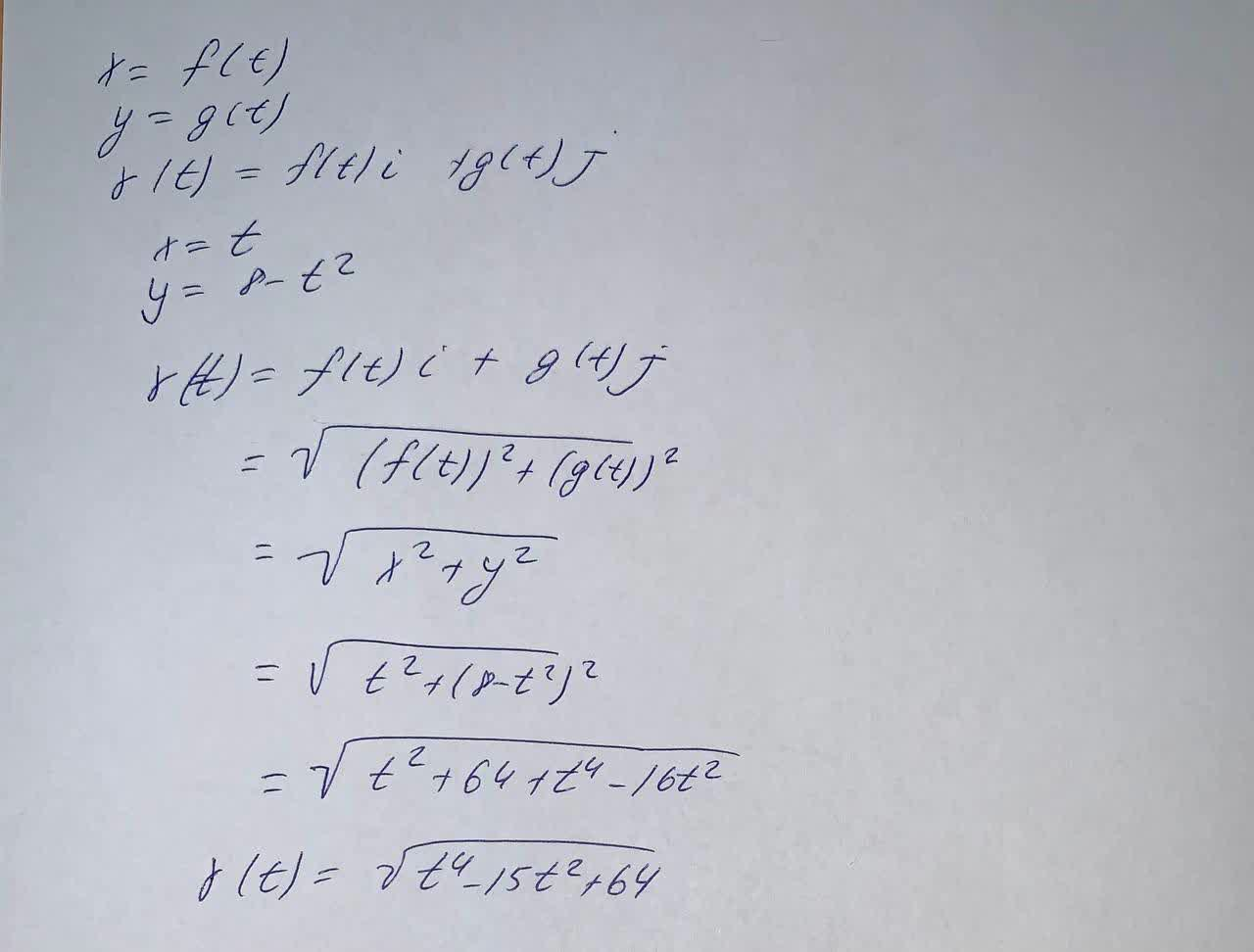# A vector-valued function r(t) with its defining parametric equations is given by the following.x = f(t)y = g(t)r(t) = ft(i) + g(t)jNow find the vector-valued function r(t) with the given parametric equations.Zoe Oneal 2021-09-13 Answered

A vector-valued function $r\left(t\right)$ with its defining parametric equations is given by the following.
$x=f\left(t\right)$
$y=g\left(t\right)$
$r\left(t\right)=ft\left(i\right)+g\left(t\right)j$
Now find the vector-valued function $r\left(t\right)$ with the given parametric equations.

You can still ask an expert for help

• Questions are typically answered in as fast as 30 minutes

Solve your problem for the price of one coffee

• Math expert for every subject
• Pay only if we can solve itottcomn

A vector-valued function r(t) with its defining parametric equations is given by the following